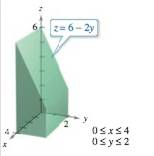Chapter 14.2, Problem 22E

Chapter
Section
Textbook Problem

Finding Volume In Exercises 21-26, use a double integral to find the volume of the indicated solid.To determine

To calculate: The volume of the solid given below.Explanation

Given:

The provided solid is:

Formula used:

The volume of a solid is provided by,

V=Rf(x,y)dA

The integral of, xndx=xn+1n+1+c

Calculation:

The volume of a solid is provided by,

V=Rf(x,y)dA

Where, f(x,y)=z

From the figure,

f(x,y)=z=62y, x goes from 0 to 4 and y goes from 0 to 2.

The projected view can be sketched on x-y plane as,

Still sussing out bartleby?

Check out a sample textbook solution.

See a sample solution

The Solution to Your Study Problems

Bartleby provides explanations to thousands of textbook problems written by our experts, many with advanced degrees!

Get Started

Solve the equations in Exercises 126. 14x2=0

Finite Mathematics and Applied Calculus (MindTap Course List)

In Exercises 1-6, simplify the expression. (36)2+[ 1(5) ]2

Calculus: An Applied Approach (MindTap Course List)

In Exercises 107-120, factor each expression completely. 120. x3 27

Applied Calculus for the Managerial, Life, and Social Sciences: A Brief Approach

For , f′(x) =

Study Guide for Stewart's Single Variable Calculus: Early Transcendentals, 8th